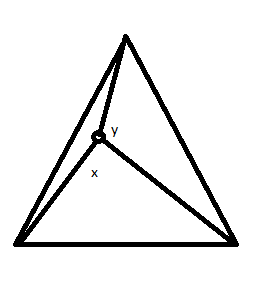BrainDen.com - Brain Teasers
• 0

## Question

Suppose you have a point within a equilateral triangle. If you were to connect each vertex to this point you would make three new line segments. Assume that you knew two of the angles formed at the point. Build a triangle out of these line segments.  What can the two known angles tell us about the angles of this newly created triangle.## Recommended Posts

• 1

Nice problem. I'll get it started by observing that no matter where the point in placed,

Spoiler

The three line segment lengths { li, lj, lk } obey the inequality li + lj  >=  a  >=  lk , for all i, j, k, where a is the length of a triangle side. The line segments therefore always can form a triangle.

## Join the conversation

You can post now and register later. If you have an account, sign in now to post with your account.×   Pasted as rich text.   Paste as plain text instead

Only 75 emoji are allowed.

×   Your previous content has been restored.   Clear editor

×   You cannot paste images directly. Upload or insert images from URL.

×